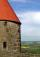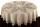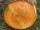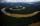# Volcano

The crater of a volcano is approximately in the shape of a cone of a base 3.1416 sq. Mi. The crater's depth is 1500 ft. How many cubic yards of earth would be required to fill this cavity?

Result

x =  1621903360 yd3

#### Solution:Leave us a comment of example and its solution (i.e. if it is still somewhat unclear...):

Showing 0 comments:Be the first to comment!#### To solve this example are needed these knowledge from mathematics:

Do you want to convert area units? Do you know the volume and unit volume, and want to convert volume units?

## Next similar examples:

1. Volume of coneFind the volume of a right circular cone-shaped building with a height of 9 cm and a radius base of 7 cm.
2. CableCable consists of 8 strands, each strand consists of 12 wires with diameter d = 0.5 mm. Calculate the cross-section of the cable.
3. Pipe cross sectionThe pipe has an outside diameter 1100 mm and the pipe wall is 100 mm thick. Calculate the cross section of this pipe.
4. Round table tableclothRound table has area 50.24 dm2. Calculate the diameter of the circular tablecloth, if it is extend beyond the edge of the table by 30 cm.
5. DiameterWhat is the inside diameter of the cylinder container and if half a liter of water reaches a height 15 cm?
6. ConvaHow many liters of water fit into the shape of a cylinder with a bottom diameter 20 cm and a height 45 cm?
7. A trapezoidA trapezoid 75 ft wide on top 85 ft on the bottom, the height is 120 ft. What is its area in the square yds?
8. SawdustHow many cubic centimeters of wood sawdust is created by cut the tree trunk with a diameter of 66 cm and when the gap width is 5 mm?
9. Truncated coneCalculate the volume of a truncated cone with base radiuses r1=13 cm, r2 = 10 cm and height v = 8 cm.
10. Rotating coneCalculate volume of a rotating cone with base radius r=12 cm and height h=7 cm.
11. Ice cream in coneIn the ice cream cone with a diameter of 5.2 cm is 1.3 dl of ice cream. Calculate the depth of the cone.
12. Reservoir 3How many cubic feet of water is stored in the reservoir that has a length of 200 feet, a width of 100 feet, an overflow depth of 32 feet, and a current water level of 24 feet?
13. The potDiameter of the pot 38 cm. The height is 30 cm. How many liters of water can fit in the pot?
14. TroughHow many liters of water per second can go via trough, which has a cross section of semicircle with radius 2.5 m and speed of water is 147 cm per second?
15. Common cylinderI've quite common example of a rotary cylinder. Known: S1 = 1 m2, r = 0.1 m Calculate : v =? V =? You can verify the results?
16. Railway wagonThe railway wagon holds 75 m3 load. Wagon can carry a maximum weight of 30 tonnes. What is the maximum density that may have material with which we could fill this whole wagon? b) what amount of peat (density 350 kg/m3) can carry 15 wagons?
17. A pipeA radius of a cylindrical pipe is 2 ft. If the pipe is 17 ft long, what is its volume?